# RD Sharma Solutions for Class 8 Math Chapter 3 - Squares and Square Roots (Part-5) Notes | Study RD Sharma Solutions for Class 8 Mathematics - Class 8

## Class 8: RD Sharma Solutions for Class 8 Math Chapter 3 - Squares and Square Roots (Part-5) Notes | Study RD Sharma Solutions for Class 8 Mathematics - Class 8

The document RD Sharma Solutions for Class 8 Math Chapter 3 - Squares and Square Roots (Part-5) Notes | Study RD Sharma Solutions for Class 8 Mathematics - Class 8 is a part of the Class 8 Course RD Sharma Solutions for Class 8 Mathematics.
All you need of Class 8 at this link: Class 8

#### (i) 441/961(ii) 324/841(iii)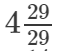(iv)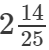(v)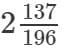(vi)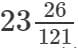(vii)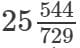(viii)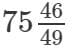(ix)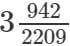(x)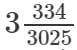(xi)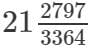(xii)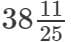(xiii)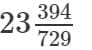(xiv)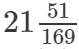(xv)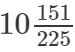#### Answer 1: (i) We know: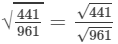Now, let us compute the square roots of the numerator and the denominator separately.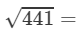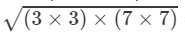=3×7=21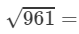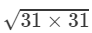=31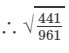=
21/31
(ii)We know: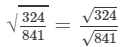Now, let us compute the square roots of the numerator and the denominator separately.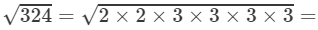2×3×3=18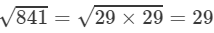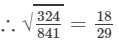(iii) By looking at the book's answer key, the fraction should be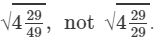We know: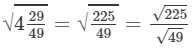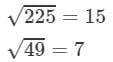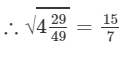(iv) We know: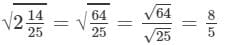(v) We know: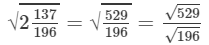Now, let us compute the square roots of the numerator and the denominator separately.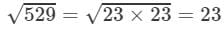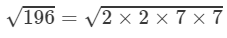=2×7=14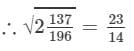(vi) We know: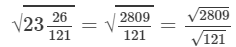Now, let us compute the square roots of the numerator and the denominator separately.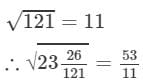(vii) We know: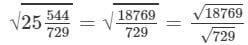Now, let us compute the square roots of the numerator and the denominator separately.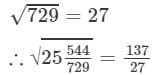(viii) We know: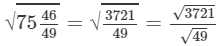Now, let us compute the square roots of the numerator and the denominator separately.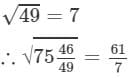(ix) We know: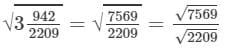Now, let us compute the square roots of the numerator and the denominator separately.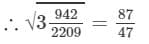(x) We know: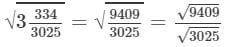Now, let us compute the square roots of the numerator and the denominator separately.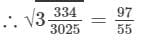(xi) We know: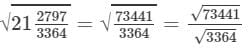Now, let us compute the square roots of the numerator and the denominator separately.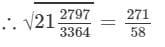(xii) We know: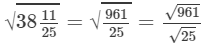Now, let us compute the square roots of the numerator and the denominator separately.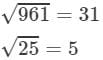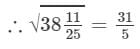(xiii) We know: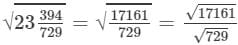Now, let us compute the square roots of the numerator and the denominator separately.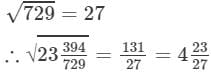(xiv) We know: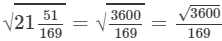Now, let us compute the square roots of the numerator and the denominator separately.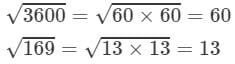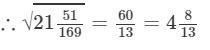(xv) We know: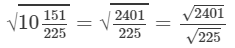Now let us compute the square roots of the numerator and the denominator separately.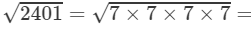7×7=49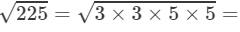3×5=15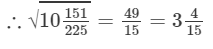#### Question 2: Find the value of: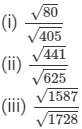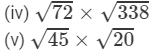#### (ii) Computing the square roots: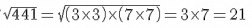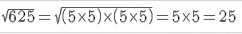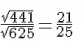#### (iii) We have: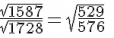(by dividing both numbers by 3)Computing the square roots of the numerator and the denominator: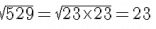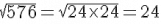∴∴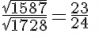#### (iv) We have: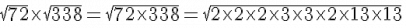=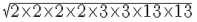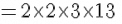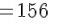#### (v) We have: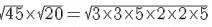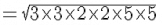= 30

#### Answer 3: The length of one side is the square root of the area of the field. Hence, we need to calculate the value of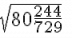We have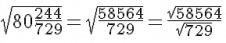Now, to calculate the square root of the numerator and the denominator: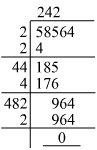We know that: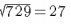#### Hence, the length of one side of the playground is 156 metres.

The document RD Sharma Solutions for Class 8 Math Chapter 3 - Squares and Square Roots (Part-5) Notes | Study RD Sharma Solutions for Class 8 Mathematics - Class 8 is a part of the Class 8 Course RD Sharma Solutions for Class 8 Mathematics.
All you need of Class 8 at this link: Class 8Use Code STAYHOME200 and get INR 200 additional OFF

## RD Sharma Solutions for Class 8 Mathematics

88 docs

Track your progress, build streaks, highlight & save important lessons and more!

,

,

,

,

,

,

,

,

,

,

,

,

,

,

,

,

,

,

,

,

,

;